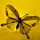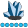## Value calculations

`data Exposure;input Hospital \$ Bug \$ Drug \$ it_n ps_n ns_n;datalines;ABC Ecoli MC 22 0.5 11ABC EcoliES MC 13 0.7 9.1XYZ Kleb MN 100 0.6 60XYZ KlebES MN 33 1 33RTY Ecoli FL 50 0.4 20RTY EcoliES FL 20 0.1 2;;;run; `

Hi,

I have the above data sample. The data contains the following variables Hospital, drug, bug, it_n (number of tests, ps_n (%susceptibility) ns_n (number of susceptible tests).

Some hospitals as in the dataset have values for 2 bugs instead of one,  we need to keep values for Ecoli and Kleb and remove EcoliES and KlebES based on this formula:  Total number of tests susceptible to Drug/ Total number of tests, for example, we'll end up with Ecoli only and calculate the values as (11 + 9.1 / 22+ 13) = 60%, so 0.6 for ps_n 20.1  for ns_n and 35 for it_n.

Is there a way to do this in SAS?

Thank you,

Razina

2 REPLIES 2whymath
Lapis Lazuli | Level 10

## Re: Value calculations

You may want a DOW-Loop way:

``````data want;
do until(last.drug);
set exposure;
by hospital drug notsorted;
sum_it_n=sum(sum_it_n,it_n);
sum_ns_n=sum(sum_ns_n,ns_n);
end;

do until(first.drug);
set exposure;
by hospital drug notsorted;
it_n=sum_it_n;
ns_n=sum_ns_n;
ps_n=ns_n/it_n;
end;
run;``````

## Re: Value calculations

Your sample data leaves a lot to presume.  I presume that

1. Each hospital either has just one value for bug, or two values for bug, (one of the form xxxES and the other of the form xxx).
2. Each hospital has a constant value for DRUG.
3. You want one record per hospital with
1. aggregate counts (it_n and ns_n)
2. recalculated ps_n
3. a bug value without the trailing "ES".
4. The data are already grouped by hospital.

``````data Exposure;
input Hospital \$ Bug \$ Drug \$ it_n ps_n ns_n;
datalines;
ABC Ecoli   MC  22 0.5 11
ABC EcoliES MC  13 0.7  9.1
XYZ Kleb    MN 100 0.6 60
XYZ KlebES  MN  33 1   33
RTY Ecoli   FL  50 0.4 20
RTY EcoliES FL  20 0.1  2
run;

data want (drop=sum_:);
set exposure;
by hospital notsorted;
if first.hospital then call missing(sum_itn,sum_nsn);
sum_itn+it_n;
sum_nsn+ns_n;
if last.hospital;
it_n=sum_itn;
ns_n=sum_nsn;
ps_n=ns_n/it_n;
if find(cats(bug,'!'),'ES!') then bug=substr(bug,1,length(bug)-2);
run;``````

--------------------------
The hash OUTPUT method will overwrite a SAS data set, but not append. That can be costly. Consider voting for Add a HASH object method which would append a hash object to an existing SAS data set

Would enabling PROC SORT to simultaneously output multiple datasets be useful? Then vote for
Allow PROC SORT to output multiple datasets

--------------------------
Discussion stats
• 2 replies
• 175 views
• 0 likes
• 3 in conversation